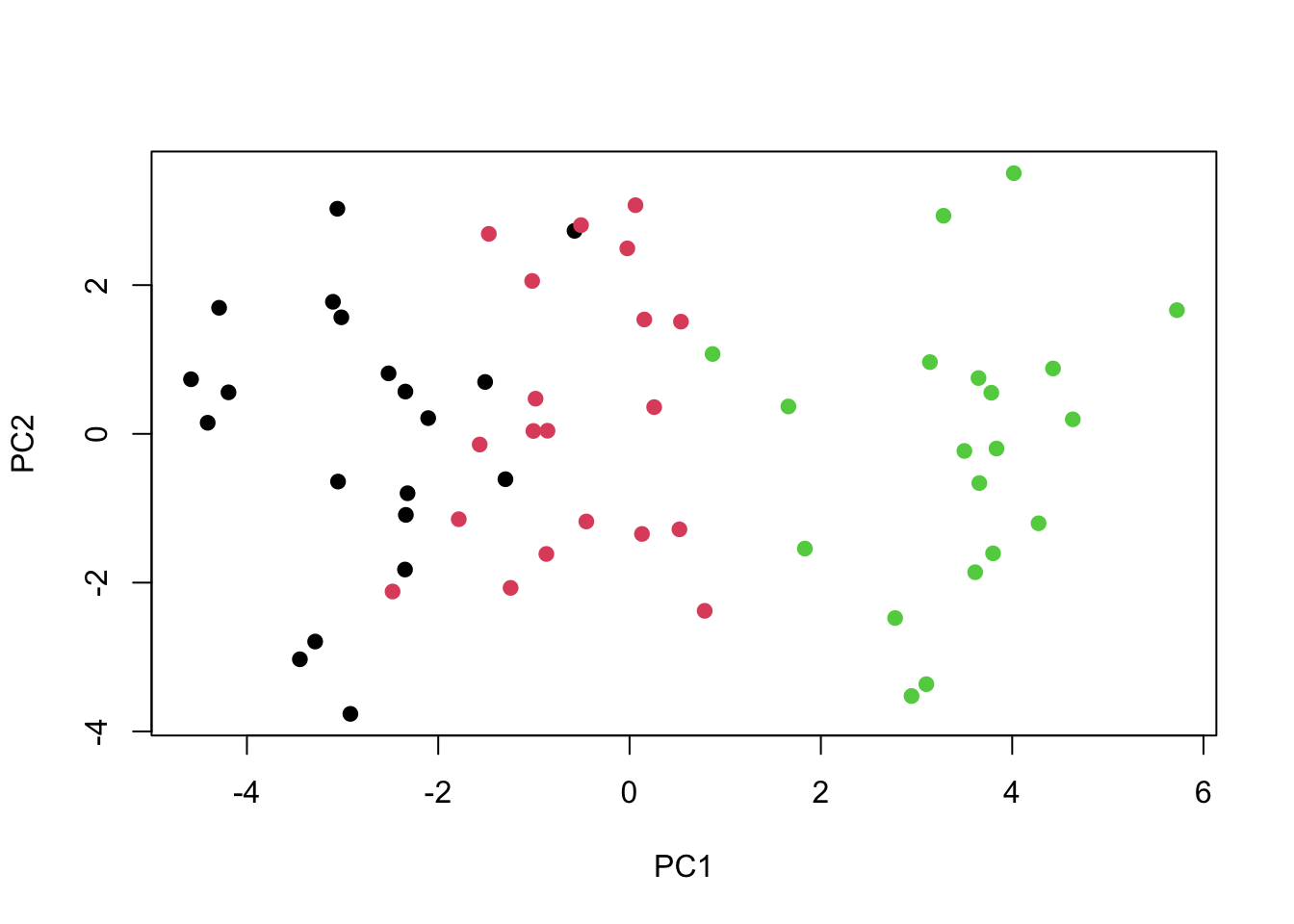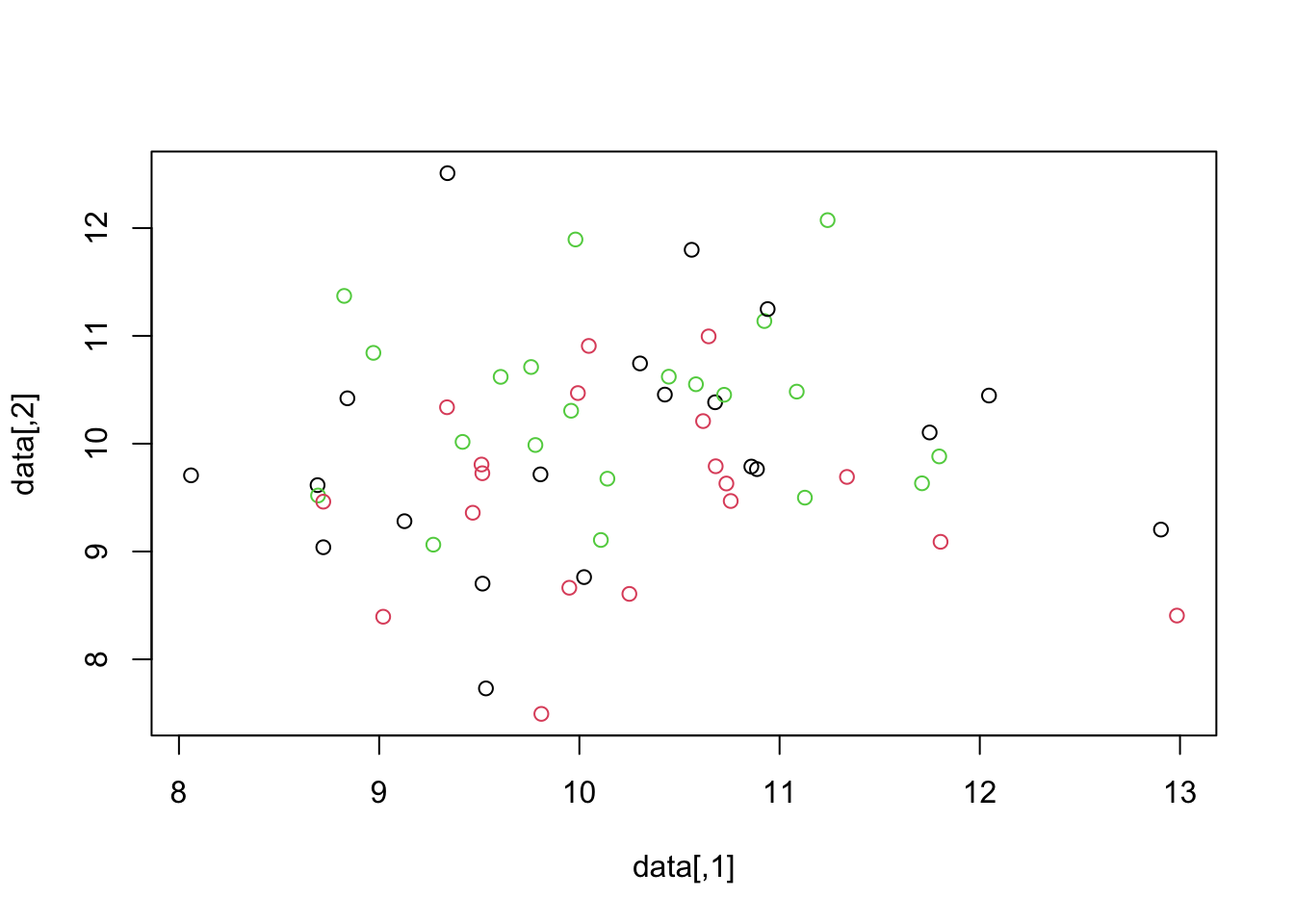ISLR Home

# Question

In this problem, you will generate simulated data, and then perform PCA and K-means clustering on the data.

1. Generate a simulated data set with 20 observations in each of three classes (i.e. 60 observations total), and 50 variables. Hint: There are a number of functions in R that you can use to generate data. One example is the rnorm() function; runif() is another option. Be sure to add a mean shift to the observations in each class so that there are three distinct classes.
2. Perform PCA on the 60 observations and plot the first two prin- cipal component score vectors. Use a different color to indicate the observations in each of the three classes. If the three classes appear separated in this plot, then continue on to part (c). If not, then return to part (a) and modify the simulation so that there is greater separation between the three classes. Do not continue to part (c) until the three classes show at least some separation in the first two principal component score vectors.
3. Perform K-means clustering of the observations with K = 3. How well do the clusters that you obtained in K-means cluster- ing compare to the true class labels? Hint: You can use the table() function in R to compare the true class labels to the class labels obtained by clustering. Be careful how you interpret the results: K-means clustering will arbitrarily number the clusters, so you cannot simply check whether the true class labels and clustering labels are the same.
4. Perform K-means clustering with K = 2. Describe your results.
5. Now perform K-means clustering with K = 4, and describe your results.
6. Now perform K-means clustering with K = 3 on the first two principal component score vectors, rather than on the raw data. That is, perform K-means clustering on the 60 × 2 matrix of which the first column is the first principal component score vector, and the second column is the second principal component score vector. Comment on the results.
7. Using the scale() function, perform K-means clustering with K = 3 on the data after scaling each variable to have standard deviation one. How do these results compare to those obtained in (b)? Explain.

``````library(ISLR)
library(tidyverse)``````

# 10a Generate Sample Data

Generate a simulated data set with 20 observations in each of three classes (i.e. 60 observations total), and 50 variables.

Hint: There are a number of functions in R that you can use to generate data. One example is the rnorm() function; runif() is another option. Be sure to add a mean shift to the observations in each class so that there are three distinct classes.

``````# From Lab 02
# x=matrix(rnorm(50*2), ncol=2)
set.seed(2)
x0 = matrix(rnorm(20*3*50, mean=sqrt(runif(1)), sd=0.1), ncol=50) # 0.001
x = x0
x[1:20, 2] = 1
x[21:40, 1] = 2
x[21:40, 2] = 2
x[41:60, 1] = 1``````
``````data = matrix(sapply(1:3,function(x){ rnorm(20*50, mean = 10*sqrt(x))  }),ncol=50)    # 20 obs. in each class with 50 features.
dim(data)``````
``##  60 50``
``````class=unlist(lapply(1:3,function(x){rep(x,20)}))
class``````
``````##   1 1 1 1 1 1 1 1 1 1 1 1 1 1 1 1 1 1 1 1 2 2 2 2 2 2 2 2 2 2 2 2 2 2 2 2 2 2
##  2 2 3 3 3 3 3 3 3 3 3 3 3 3 3 3 3 3 3 3 3 3``````

# 10b Plot first two principal component score vectors

Perform PCA on the 60 observations and plot the first two principal component score vectors. Use a different color to indicate the observations in each of the three classes. If the three classes appear separated in this plot, then continue on to part (c). If not, then return to part (a) and modify the simulation so that there is greater separation between the three classes. Do not continue to part (c) until the three classes show at least some separation in the first two principal component score vectors.

``````pr.out=prcomp(data, scale=FALSE) # Don't need scale because mean=0, sd=0.001
#summary(pr.out)
#plot(pr.out\$x[,c(1,2)],col=class)``````
``````#plot(pr.out\$x[,c(1,2)], col=c(1,2,3))
#plot(pr.out\$x[,1:2], col=2:4, xlab="Z1", ylab="Z2", pch=19)
plot(pr.out\$x[,c(1,2)],col=class, pch=19)``````# 10c K-means clustering, K = 3.

Perform K-means clustering of the observations with K = 3. How well do the clusters that you obtained in K-means clustering compare to the true class labels?

Hint: You can use the table() function in R to compare the true class labels to the class labels obtained by clustering. Be careful how you interpret the results: K-means clustering will arbitrarily number the clusters, so you cannot simply check whether the true class labels and clustering labels are the same.

``````km.out=kmeans(data,3,nstart=20)
km.out\$cluster``````
``````##   1 1 3 1 1 1 1 1 1 3 1 1 3 3 1 1 1 1 1 1 3 3 3 3 3 3 1 3 3 3 3 3 3 1 1 3 3 3
##  3 1 2 2 2 3 2 2 2 2 2 2 2 2 2 2 2 2 2 2 2 2``````
``table(km.out\$cluster)``
``````##
##  1  2  3
## 20 19 21``````
``````#class_vars = c(rep(1,20), rep(2,20), rep(3,20))
table(km.out\$cluster, class)``````
``````##    class
##      1  2  3
##   1 16  4  0
##   2  0  0 19
##   3  4 16  1``````
``````# plot(pr, col=(km.out\$cluster + 1), main="K-Means Clustering Results with K=3", xlab="", ylab="", pch=20, cex=2)
plot(data, col=km.out\$cluster)``````# 10d K-means clustering, K = 2

Perform K-means clustering with K = 2. Describe your results

``````km.out=kmeans(data,2,nstart=20)
km.out\$cluster``````
``````##   2 2 2 2 2 2 2 2 2 2 2 2 2 2 2 2 2 2 2 2 2 2 2 2 2 1 2 2 2 2 2 2 2 2 2 2 2 2
##  2 2 1 1 1 1 1 1 1 1 1 1 1 1 1 1 1 1 1 1 1 1``````
``table(km.out\$cluster)``
``````##
##  1  2
## 21 39``````
``table(km.out\$cluster, class)``
``````##    class
##      1  2  3
##   1  0  1 20
##   2 20 19  0``````

41 misclassified (20+1+18+2)

# 10e K-means clustering, K = 4

Now perform K-means clustering with K = 4, and describe your results

``````km.out=kmeans(data,4,nstart=20)
km.out\$cluster``````
``````##   1 2 1 1 2 2 2 2 1 1 2 2 4 4 2 2 1 1 1 2 1 1 4 4 1 4 1 4 4 4 4 4 1 1 1 4 4 4
##  1 2 3 3 3 3 3 3 3 3 3 3 3 3 3 3 3 3 3 3 3 3``````
``table(km.out\$cluster)``
``````##
##  1  2  3  4
## 16 11 20 13``````
``table(km.out\$cluster, class)``
``````##    class
##      1  2  3
##   1  8  8  0
##   2 10  1  0
##   3  0  0 20
##   4  2 11  0``````

# 10f K-means clustering, K = 3; 2 PCA

Now perform K-means clustering with K = 3 on the first two principal component score vectors, rather than on the raw data. That is, perform K-means clustering on the 60 × 2 matrix of which the first column is the first principal component score vector, and the second column is the second principal component score vector. Comment on the results.

``#summary(pr.out)``
``````km.out=kmeans(pr.out\$x[,1:2],3,nstart=20)
km.out\$cluster``````
``````##   2 1 2 1 1 2 1 1 2 2 1 1 1 2 1 1 1 2 2 1 2 1 1 1 2 2 2 1 1 1 1 1 2 1 2 2 1 1
##  2 1 3 3 3 3 3 3 3 3 3 3 1 3 3 3 3 3 3 3 3 3``````
``table(km.out\$cluster)``
``````##
##  1  2  3
## 25 16 19``````
``table(km.out\$cluster, class)``
``````##    class
##      1  2  3
##   1 12 12  1
##   2  8  8  0
##   3  0  0 19``````

# 10g Scale, K-means clustering, K = 3

Using the scale() function, perform K-means clustering with K = 3 on the data after scaling each variable to have standard deviation one. How do these results compare to those obtained in (b)? Explain.

``````sc = scale(data)
km.out = kmeans(sc, 3, nstart = 20)
km.out\$cluster``````
``````##   3 3 2 1 3 3 1 1 3 2 1 3 1 2 3 3 3 3 1 1 2 3 1 1 3 2 3 3 1 1 3 1 2 3 3 2 1 1
##  3 3 2 2 1 1 2 3 2 2 2 2 1 1 2 2 2 1 2 1 2 1``````
``plot(data, col=km.out\$cluster)``